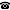# 2020 seminar talk: Generalized Cardinal Characteristics for Sets of Functions

Talk held by Corey Bacal Switzer (City University of New York, Graduate Center, USA) at the KGRC seminar on 2020-01-16.

### Abstract

Cardinal characteristics on the generalized Baire and Cantor spaces $\kappa^\kappa$ and $2^\kappa$ have recently generated significant interest. In this talk I will introduce a different generalization of cardinal characteristics, namely to the space of functions $f:\omega^\omega \to \omega^\omega$. Given an ideal $\mathcal I$ on Baire space and a relation $R$ let us define $f R_{\mathcal I} g$ for $f$ and $g$ functions from $\omega^\omega$ to $\omega^\omega$ if and only if $f(x) R g(x)$ for an $\mathcal I$-measure one set of $x \in \omega^\omega$. By letting $\mathcal I$ vary over the null ideal, the meager ideal and the bounded ideal; and $R$ vary over the relations $\leq^*$, $\neq^*$ and $\in^*$ we get 18 new cardinal characteristics by considering the bounding and dominating numbers for these relations. These new cardinals form a diagram of provable implications similar to the Cichoń diagram. They also interact in several surprising ways with the cardinal characteristics on $\omega$. For instance, they can be arbitrarily large in models of CH, yet they can be $\aleph_1$ in models where the continuum is arbitrarily large. They are bigger in the Sacks model than the Cohen model. I will introduce these cardinals, show some of the provable implications and discuss what is known about consistent inequalities, including new generalizations of well-known forcing notions on the reals to this context. This includes joint work with Jörg Brendle.

Kurt Gödel Research Center for Mathematical Logic. Währinger Straße 25, 1090 Wien, Austria.+43-1-4277-50501. Last updated: 2010-12-16, 04:37.